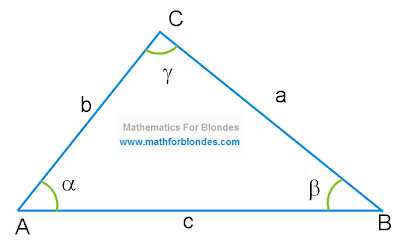## 1.30.2011

### Triangle

The triangle is the elementary polygon. Such definition of a triangle is given by Wikipedia. We will not argue with collective reason, we will try to judge by own strength. The triangle is a geometrical figure which consists of three tops and three parties. As appears from the name "triangle", this geometrical figure has three corners. How the triangle looks? Here a triangle photo in all its beauty.Triangle

Despite such simple-minded appearance of a triangle, I very much doubt correctness of the statement of Wikipedia that the triangle is the elementary polygon. It is too much at a triangle of any gadgets. Has specially looked at the mathematical directory and here acknowledgement of my words: to a triangle it is devoted 6 (six!) the pages, to all quadrangles together taken, only 5 (five) pages. External simplicity of a triangle at all does not mean simplicity mathematical.

And so, we will start to assort a triangle on stones. Three points which are not lying on one straight line, form a triangle. These points are called as triangle tops. The request not to confuse to mountain tops is absolutely another. The triangle has three tops which are designated by the big Latin letters A, B, C (it is a surname at tops such). You ask, than these Latin letters differ from Russian letters And, In, With? A family tree at these letters different, and consequently also an order of an arrangement of these letters in the alphabet.

Between triangle tops there are triangle parties. These are such equal paths on which it is possible to run across from one top of a triangle to other top. The triangle parties also are inviolable frontiers of a triangle. In these borders there is a triangle area. Everything that is outside behind these borders, into the triangle area does not enter. Along these borders frontier guards with dogs go and watch, that another's area has not got into the triangle area, and the triangle area has not run away abroad from such good life in a triangle. The triangle parties by small Latin letters a, b, c are designated.

Just the same small Latin letters a, b, c the length of these parties of a triangle is designated. The strict boundary heads after all need to know, how many kilometres poor boundary dogs have run? How many kilometres passed unfortunate frontier guards are, for some reason, chiefs never interests. When speech comes about length of the parties of a triangle, probably other designation - two big Latin letters with two vertical sticks: |AB |, |BC |, |AC |. In this case the designation of length of the party of a triangle undertakes on a surname of tops of a triangle between which there is this party (look a photo triangle). In full conformity with rules of mathematical bureaucracy it is possible to write down:

a = |BC|

b = |AC|

c = |AB|

From here the first law of a triangle for blondes is very easily deduced: the length of the party of a triangle is designated by two big Latin буковками or one small буковкой, that which is not present among big буковок.

It is very logical to assume that triangle corners also have the designations. Each corner of a triangle has cosy settled down in triangle top between two parties. Designate triangle corners small letters of the Greek alphabet α (alpha), β (beta), γ (gamma). The sum of all corners of a triangle is equal 180 degrees. Such to itself democracy of a triangle: if you a corner also want to be more, select at other corner and use. All as in life. Therefore corners in a triangle meet the different: stupid and pompous (you such perfectly know) near to thin and graceful (blondes). Democracy of a triangle in the mathematician is called "the triangle Theorem" and it sounds so: the sum of all corners of a triangle equals hundred eighty degrees. In mathematical symbols the triangle theorem looks so:

α + β + γ = 180°

Depending on a kind of the corners which have formed Open Company "Triangle Ltd.", triangles differ on appearance. It is such geometrical dress-code for triangles. But about it we will talk next time.

Here you will find answers for such questions: the sum of all corners on a triangle.